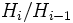# Finite normal series operator

This article defines a group property modifier (a unary group property operator) -- viz an operator that takes as input a group property and outputs a group property

## Definition

### Property-theoretic definition

The finite normal series operator is a group property modifier that takes as input a group property$p$ and outputs the Kleene star closure of$p$ with respect to the group extension operator, right-bracketed.

### Definition with symbols

Given a group property$p$, the finite normal series operator applied to$p$ returns the group property$q$ defined as follows: a group$G$ has property$q$ if there is a finite normal series$e = H_0 \le H_1 \le \ldots \le H_r = G$ such that each$H_i$ is normal in$G$ and such that$H_i/H_{i-1}$ satisfies property$p$ for every$i$.

## Metaproperties

### Monotonicity

This group property modifier is monotone, viz if$p \le q$ are group properties and$f$ is the operator, then$f(p) \le f(q)$

If$p \le q$ are group properties, then the image of the finite normal series operator on$p$ implies the image of the finite normal series operator on$q$.

### Ascendance

This group property modifier is ascendant, viz the image of any group property under this modifier is always weaker than the group property we started with

For any group property$p$, any group satisfying$p$ also satisfies the image of$p$ under the finite normal series operator.

### Idempotence

The finite normal series operator need not be idempotent.